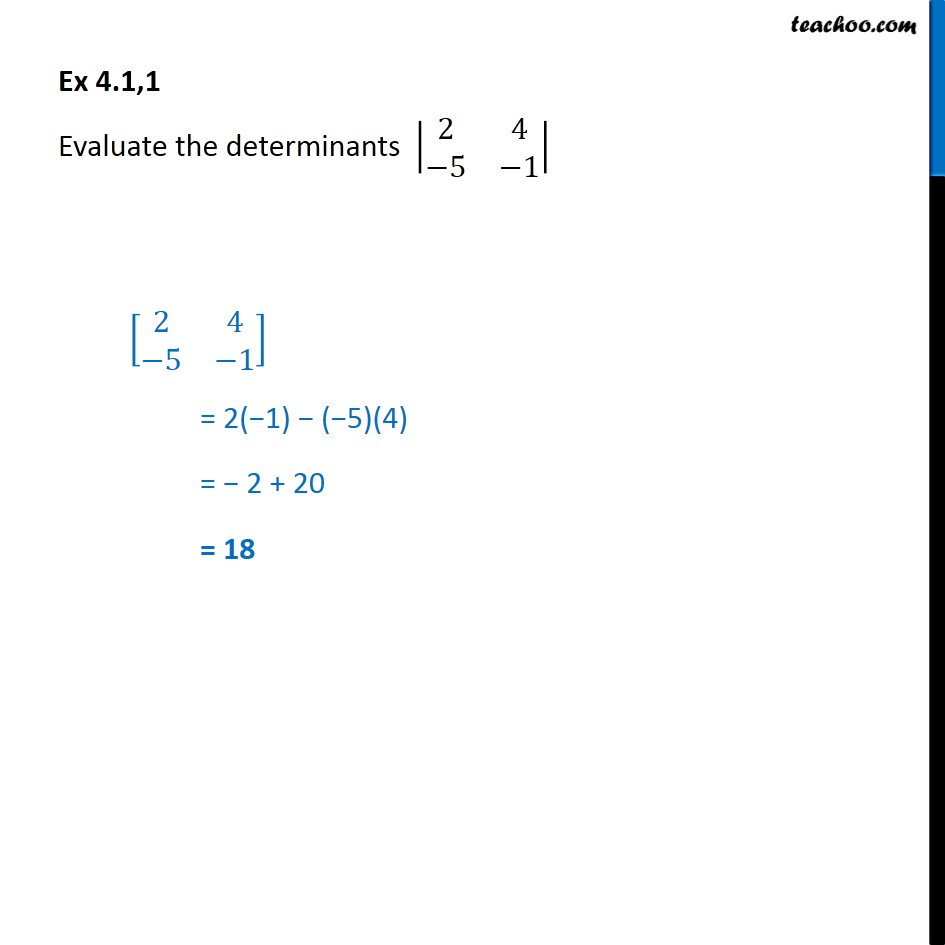Subscribe to our Youtube Channel - https://you.tube/teachoo

1. Chapter 4 Class 12 Determinants
2. Concept wise
3. Finding determinant of a 2x2 matrix

Transcript

Ex 4.1, 1 - Chapter 4 Class 12 Determinants - NCERT Book Evaluate the determinant | 2 4 -5 -1| | 2 4 -5 -1| = 2(−1) − (−5)(4) = − 2 + 20 = 18

Finding determinant of a 2x2 matrix